# 3-9.The 6 Fundamental law and the 4 thermodynamic law about drying.

### The 0th law of thermodynamics—Law of heat balances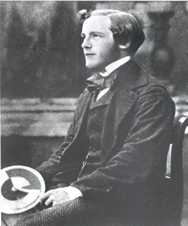James Clerk Maxwell FRS FRSE (13 June 1831 – 5 November 1879) Scottish mathematical physicist.

If the objects A and B, B and C are thermal balances, A and C are also in a thermal balance.

AT=BT, BT=CT ⇒ AT=CT

The law is important for the mathematical formulation of thermodynamics, which needs the assertion that the relation of thermal equilibrium is an equivalence relation. This information is needed for the mathematical definition of temperature that will agree with the physical existence of valid thermometers.

### The 1th law of thermodynamics— Law of conservation of energy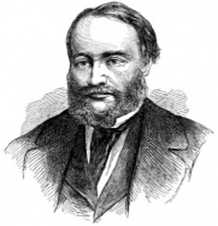James Prescott Joule FRS (24 December 1818 – 11 October 1889) English physicist and brewer.,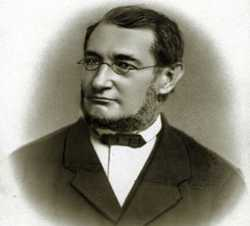Julius Robert von Mayer (November 25, 1814 – March 20, 1878) German physician and physicist.

It is von Meyer and Jules have found the law of conservation of energy (first law of thermodynamics) individually in the same period.

Given the heat of ΔQ only in closed systems, and the rest are stored as internal energy ΔU by the work of ΔW with respect to the external.

ΔQ=ΔW+ΔU

ΔQ:Amount of heat given to the system
ΔW:Work that has been removed from the system
ΔU:Increase the amount of internal energy U of the system

### The 2th law of thermodynamics—Law of irreversibility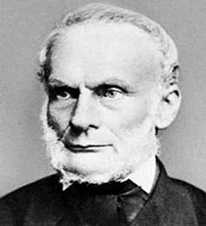Rudolf Julius Emmanuel Clausius, ( 2 Jan 1822 – 24 August 1888 ) German physician and physicist.

Clausius presented his paper “On a Modified Form of the Second Fundamental Theorem in the Mechanical Theory of Heat.”in 1854,
He has established the second law of thermodynamics.

“Heat tended to eliminate the temperature difference is always, therefore. To move to the cold object from the hot object is always”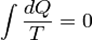Q:Amount of heat that is generated from work
T:Temperature

### The 3th law of thermodynamics— Entropy becomes zero at absolute zero.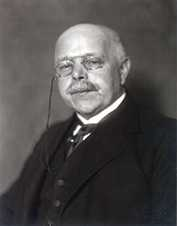Walther Hermann Nernst (25 June 1864 – 18 November 1941) German physicist
1920 Nobel Prize in chemistry.

Nernst researched osmotic pressure and electrochemistry. In 1905, he established what he referred to as his “New Heat Theorem”, later known as the Third law of thermodynamics (which describes the behavior of matter as temperatures approach absolute zero). This is the work for which he is best remembered, as it provided a means of determining free energies (and therefore equilibrium points) of chemical reactions from heat measurements. Theodore Richards claimed Nernst had stolen the idea from him, but Nernst is almost universally credited with the discovery.

Entropy becomes zero at absolute zero.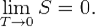### Fourier’s law — Heat conduction equation — Heat flux density is proportional to the temperature gradient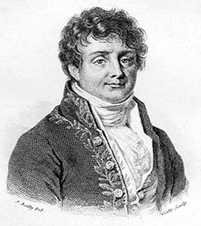Jean Baptiste Joseph Fourier, Baron de. (21 March 1768 – 16 May 1830) French mathematician and physicist

In 1812, Won an Academy Award and submit the “The Analytical Theory of Heat” paper
In 1822 Fourier published his work on heat flow in Théorie analytique de la chaleur (The Analytic Theory of Heat).

Heat flux density is proportional to the temperature gradient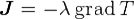J [W/m2] : Heat flow (heat flux density)
λ: Thermal conductivity
T : Temperature

λ will be expressed by the scalar if the substance is isotropic.
Generally, by an un-isotropic three-dimensional system, direction of J and grad T is not in agreement, and thermal conductivity is expressed by the tensor.

### Fick’s diffusion law — diffusion flux is proportional to the concentration gradient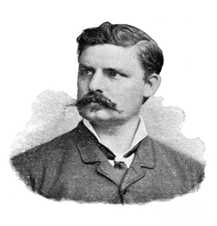Adolf Eugen Fick (3 September 1829 – 21 August 1901) German physician and physiologist.

Fick’s first law relates the diffusive flux to the concentration under the assumption of steady state.

Fick’s second law predicts how diffusion causes the concentration to change with time:

### Joule’s lawJames Prescott Joule FRS (24 December 1818 – 11 October 1889) English physicist and brewer

Joule’s first law, also known as the Joule effect, is a physical law expressing the relationship between the heat generated by the current flowing through a conductor.

Joule’s first law states that the heat Q produced when a current I flows through a resistance R for a specified time t is given by the current squared multiplied by the product of the resistance and the time, or equalitively: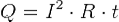Q:amount of heat produced(J)
I:current(A)
R:resistance（Ω)
t:energization time(s)

Joule’s second law states that the internal energy of an ideal gas is independent of its volume and pressure, depending only on its temperature.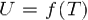U:internal energy of an ideal gas
T:temperature of the ideal gas
f:ideal gas energy function

### Combined gas law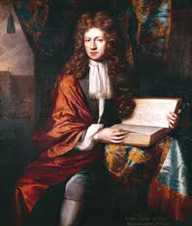Robert Boyle, FRS,Born in Lismore, (25 January 1627 – 31 December 1691) Irish natural philosopher, chemist, physicist, and inventor.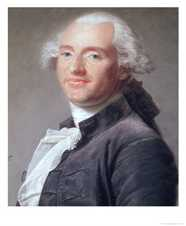Jacques Alexandre César Charles (November 12, 1746 – April 7, 1823) French inventor, scientist, mathematician, and balloonist.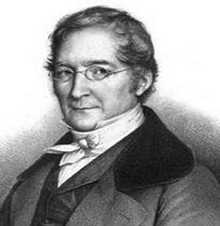Joseph Louis Gay-Lussac ( 6 December 1778 – 9 May 1850) French chemist and physicist.

The combined gas law is a gas law that combines Charles’s law, Boyle’s law, and Gay-Lussac’s law. There is no official founder for this law; it is merely an amalgamation of the three previously discovered laws. These laws each relate one thermodynamic variable to another mathematically while holding everything else constant. Charles’s law states that volume and temperature are directly proportional to each other as long as pressure is held constant. Boyle’s law asserts that pressure and volume are inversely proportional to each other at fixed temperature. Finally, Gay-Lussac’s law introduces a direct proportionality between temperature and pressure as long as it is at a constant volume. The inter-dependence of these variables is shown in the combined gas law, which clearly states that:

The ratio between the pressure-volume product and the temperature of a system remains constant.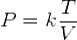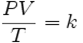P : the pressure
V : the volume
T : the temperature measured in kelvin
k : the constant (with units of energy divided by temperature).

Ideal gas of 0 ℃ will swell to twice at 273 ℃.
Ideal gas of 25 ℃ will swell to twice at 298 ℃.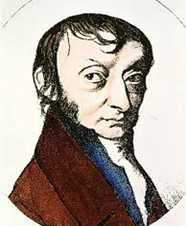Lorenzo Romano Amedeo Carlo Avogadro, conte di Quaregna e Cerreto (Torino, 9 agosto 1776 – Torino, 9 luglio 1856) Italian chemist and physicist.

For 100.00kPa and 273.15 K, the molar volume of an ideal gas is 22.712 dm3mol-1.
(Standard state:P=1.01325ｘHPa T=273.15K ≒0℃/1atm V=22.413m3)

### Newton’s law of cooling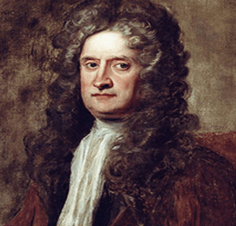Sir Isaac Newton PRS MP ( 25 December 1642 – 20 March 1727) English physicist and mathematician

Newton’s Law of Cooling states that the rate of change of the temperature of an object is proportional to the difference between its own temperature and the ambient temperature (i.e. the temperature of its surroundings).

In the medium, the rate at which heat is transferred to the medium from the solid is proportional to the temperature difference between the medium and the solid and the surface area of the solid.
The following relationships holds between amount of heat with the solid Q, time t, the surface area of the solid S, the temperature of the solid T, the temperature of the medium Tm.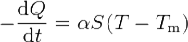Proportionality constant α is a constant determined the surface of a solid, such as by the flow and how the nature of the medium.
It called the surface thermal conductivity or heat transfer rate.